Open Access Publications from the University of California

### Recent Work

It is only in recent years that the scientific community has focused significant resources in understanding the complexity of non-linear, distributed systems involving many interacting components. While the toolbox of methods for dealing with such systems is still relatively small, tools have been developed that provide useful qualitative characterizations of the behavior of non-linear dynamical systems. Over the last thirty years advances in the theory of nonlinear dynamics have made possible the qualitative analysis of nonlinear models involving finitely many dimensions. Many of the concepts that have emerged with the development of these tools are now well-known in nonlinear science, and include chaos, strange attractors, fractals, and scaling

More recently, an emerging focus has been on methods for characterizing the qualitative behavior of high- and infinite-dimensional non-linear systems. These developments promise to put within our reach the qualitative analysis of more realistic models of the complex phenomena of interest to scientists. The power of these methods resides, in part, in the realization that the qualitative behavior of many classes of complex systems is insensitive to differences in the details of their underlying mechanisms and processes. Many apparently "different" systems exhibit qualitively similar (universality classes) of behaviours.

The purpose of the Center of Nonlinear Science (CNLS) is to make these techniques available and demonstrate to researcher in nonlinear science that, when used in conjunction with more traditional tools, these emerging methodologies are capable of providing relatively "complete" characterizations of important classes of nonlinear phenomena.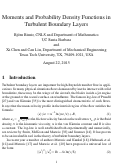## Moments and Probability Density Functions in Turbulent Boundary Layers

(2015)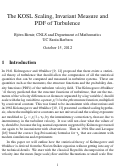## The KOSL Scaling, Invariant Measure and PDF of Turbulence

(2013)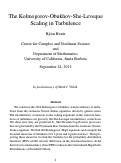## The Kolmogorov-Obukhov-She-Leveque Scaling in Turbulence

(2013)

We construct the 1962 Kolmogorov-Obukhov statistical theory of turbulence from the stochastic Navier-Stokes equations driven by generic noise. The intermittency corrections to the scaling exponents of the structure functions of turbulence are given by the She-Leveque intermittency corrections. We show how they are produced by She-Waymire log-Poisson processes, that are generated by the Feynmann-Kac formula from the stochastic Navier-Stokes equation. We find the Kolmogorov-Hopf equations and  compute the invariant measures of turbulence for 1-point and 2-point statistics. Then projecting these measures we find the formulas for the probability distribution functions (PDFs) of the velocity differences in the structure functions. In the limit of zero intermittency, these PDFs reduce to the Generalized Hyperbolic Distributions of Barndorff-Nilsen.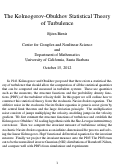## The Kolmogorov-Obukhov Statistical Theory of Turbulence

(2012)

In 1941 Kolmogorov and Obukhov proposed that there exists a statistical theory of turbulence that should allow the computation of all the statistical quantities that can be computed and measured in turbulent systems. These are quantities such as the moments, the structure functions and the probability density functions (PDFs) of the turbulent velocity field.  In this paper we will outline how to construct this statistical theory from the stochastic Navier-Stokes equation. The additive noise in the stochastic Navier-Stokes equation is generic noise given by the central limit theorem and the large deviation principle. The multiplicative noise consists of jumps multiplying the velocity, modeling jumps in the velocity gradient.We first estimate the structure functions of turbulence and establish the Kolmogorov-Obukhov {'}62 scaling hypothesis with the She-Leveque intermittency corrections. Then we compute the invariant measure of turbulence writing the stochastic Navier-Stokes equation as an infinite-dimensional Ito process and solving the linear Kolmogorov-Hopf functional differential equation for the invariant measure. Finally we project the invariant measure onto the PDF. The PDFs turn out to be the normalized inverse Gaussian (NIG) distributions of Barndorff-Nilsen, and compare well with PDFs from simulations and experiments.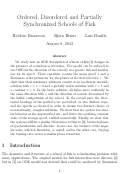## Ordered, Disordered and Partially Synchronized Schools of Fish

(2012)

We study how an ODE description of schools of fish by Birnir (2007) changes in the presence of a random acceleration. The model can be reduced to one ODE for the direction of the velocity of a generic fish and another one for its speed. These equations contain the mean speed v and a Kuramoto order parameter for the phases of the fish velocities, r. We show that their stationary solutions consist of an incoherent unstable solution with r=v=0 and a globally stable solution with r=1 and a constant v > 0. In the latter solution, all fishes move uniformly in the same direction with v and the direction of motion determined by the initial configuration of the school.In the second part, the directional headings of the particles are perturbed, in two distinct ways, and the speeds accelerated in order to obtain two distinct classes of non-stationary, complex solutions. We show that the system has similar behavior as the unperturbed one, and derive the resulting constant value of the average speed, verified numerically. Finally, we show that the system exhibits a similar bifurcation to that in Cirok and Vishek et al. 1995, between phases of synchronization and disorder. In one case, the variance of the angular noise, which is Brownian, is varied, and in the other case, varying the turning rate causes a similar phase transition.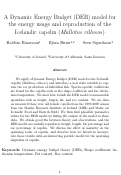## A Dynamic Energy Budget (DEB) model for the energy usage and reproduction of the Icelandic capelin (Mallotus villosus)

(2010)

We apply a Dynamic Energy Budget (DEB) model to the Icelandic capelin (Mallotus villosus) and introduce a new state variable to capture the roe production of individual fish. Species-specific coefficients are found for the capelin such as the shape-coefficient and the Arrhenius temperature. We show how to link the DEB model to measurable quantities such as weight, length, fat, and roe content. We use data on measured three year old female capelin from the 1999-2000 season from the Marine Research Institute of Iceland (MRI) and Matis, an Icelandic Food and Biotech R&D. We then find plausible parameter values for the DEB model by fitting the output of the model to these data. We obtain good fits between theory and observations, and the DEB model successfully reproduces weight, length, fat percentage and roe percentage of capelin. We discuss the effect of maturity on the spawning route of capelin, and describe how we intend to incorporate these results with an interacting particle model for the spawning migration of capelin.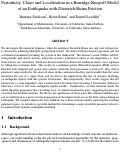## Periodicity, Chaos and Localization in a Burridge-Knopoff Model of an Earthquake with Dieterich-Ruina Friction

(2010)

We investigate the emergent dynamics when the nonlinear Dieterich-Ruina rate and state friction law is attached to a Burridge-Knopoff spring-block model. We derive both the discrete equations and the continuum formulation governing the system in this framework. The discrete system (ODEs) exhibits both periodic and chaotic motion, where the system’s transition to chaos is size-dependent, i.e. how many blocks are considered. From the discrete model we derive the nonlinear elastic wave equation by taking the continuum limit. This results in a nonlinear partial differential equation (PDE) and we find that both temporal and spatial chaos ensues when the same parameter is increased. This critical parameter value needed for the onset of chaos in the continuous model is much smaller than the value needed in the case of a single block and we discuss the implications this has on dynamic modeling of earthquake rupture with this specific friction law. Most importantly, these results suggest that the friction law is scale-dependent, thus caution should be taken when attaching a friction law derived at laboratory scales to full-scale earthquake rupture models. Furthermore, we find solutions where the initial slip pulse propagates like a traveling wave, or remains localized in space, suggesting the presence of soliton and breather solutions. We discuss the significance of these pulse-like solutions and how they can be understood as a proxy for the propagation of the rupture front across the fault surface during an earthquake. We compute analytically the conditions for soliton solutions and by exploring the resulting parameter space, we introduce a possible method for determining a range of suitable parameter values to be used in future dynamic earthquake modeling.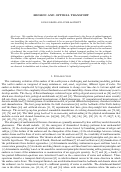## Erosion and Optimal Transport

(2010)

We consider the theory of erosion and investigate connections to the theory of optimal transport. The mathematical theory of erosion is based on two coupled nonlinear partial differential equations. The first one describing the water flow can be considered to be an averaged Navier-Stokes equation, and the second one describing the sediment flow is a very degenerate nonlinear parabolic equation. In the first half of this work, we prove existence, uniqueness, and regularity properties of weak solutions to the second model equation describing the sediment flow. This forms the basis to define an optimal transport problem for the movement of sediment; the second half of this work is devoted to this optimal transport problem for the sediment. We solve the optimal transport problem. Furthermore, we demonstrate that the optimal transport problem distinguishes a particular class of solutions to the model equation. The movement of sediment according to the solution of the optimal transport problem is identical to the movement of sediment according to these solutions of the model equation. The physical interpretation is that if the sediment flows according to the model equation on the surface of separable solutions consisting of valleys separated by mountain ridges, that are observed in simulation and in nature, then the sediment is ``optimally transported.''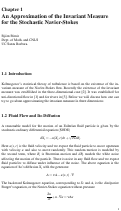## Approximation of the Invariant Measure for the Stochastic Navier-Stokes

(2009)

Kolmogorov's statistical theory of turbulence is based on the existence of the invariant measure of the Navier-Stokes flow. Recently the existence of the invariant measure was established in the three-dimensional case. It was established earlier by the author for uni-directional flow and for rivers. We discuss how one can try to go about approximating the invariant measure in three dimensions.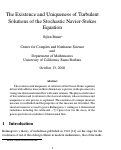## The Existence and Uniqueness of Turbulent Solutions of the Stochastic Navier-Stokes Equation

(2008)

The existence and uniqueness of solutions of the Navier-Stokes equation driven with additive noise in three dimensions is proven, in the presence of a strong uni-directional mean flow with some rotation. The physical relevance of this solution and its relation to the classical solution, whose existence and uniqueness is also proven, is explained. The existence of a unique invariant measure is established and the properties of this measure are described. The invariant measure is used to prove Kolmogorov's scaling in 3-dimensional turbulence including the celebrated -5/3 power law for the decay of the power spectrum of a turbulent 3-dimensional flow.